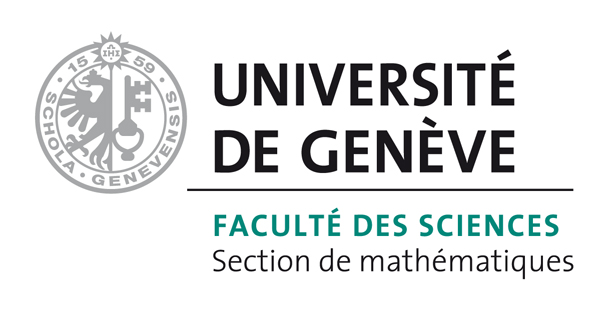# Bart Vandereycken## Subspace methods for computing the pseudospectral abscissa and the stability radius

by ,

#### Abstract:

The pseudospectral abscissa and the stability radius are well-established tools for quantifying the stability of a matrix under unstructured perturbations. Based on first-order eigenvalue expansions, Guglielmi and Overton [SIAM J. Matrix Anal. Appl., 32 (2011), pp. 1166--1192] recently proposed a linearly converging iterative method for computing the pseudospectral abscissa. In this paper, we propose to combine this method and its variants with subspace acceleration. Each extraction step computes the pseudospectral abscissa of a small rectangular matrix pencil, which is comparably cheap and guarantees monotonicity. We observe local quadratic convergence and prove local superlinear convergence of the resulting subspace methods. Moreover, these methods extend naturally to computing the stability radius. A number of numerical experiments demonstrate the robustness and efficiency of the subspace methods.

#### Reference:

D. Kressner, B. Vandereycken, "Subspace methods for computing the pseudospectral abscissa and the stability radius", In SIAM J. Matrix Anal. Appl., vol. 35, no. 1, pp. 292-313, 2014.

#### Bibtex Entry:

@article{Kressner_V_2014,
Abstract = {The pseudospectral abscissa and the stability radius are well-established tools for quantifying the stability of a matrix under unstructured perturbations. Based on first-order eigenvalue expansions, Guglielmi and Overton [SIAM J. Matrix Anal. Appl., 32 (2011), pp. 1166--1192] recently proposed a linearly converging iterative method for computing the pseudospectral abscissa. In this paper, we propose to combine this method and its variants with subspace acceleration. Each extraction step computes the pseudospectral abscissa of a small rectangular matrix pencil, which is comparably cheap and guarantees monotonicity. We observe local quadratic convergence and prove local superlinear convergence of the resulting subspace methods. Moreover, these methods extend naturally to computing the stability radius. A number of numerical experiments demonstrate the robustness and efficiency of the subspace methods.},
Author = {Kressner, D. and Vandereycken, B.},
Doi = {10.1137/120869432},
Journal = {SIAM J. Matrix Anal. Appl.},
Number = {1},
Pages = {292--313},
Pdf = {http://www.unige.ch/math/vandereycken/papers/published_Kressner_V_2014.pdf},
Title = {Subspace methods for computing the pseudospectral abscissa and the stability radius},
Volume = {35},
Year = {2014}}